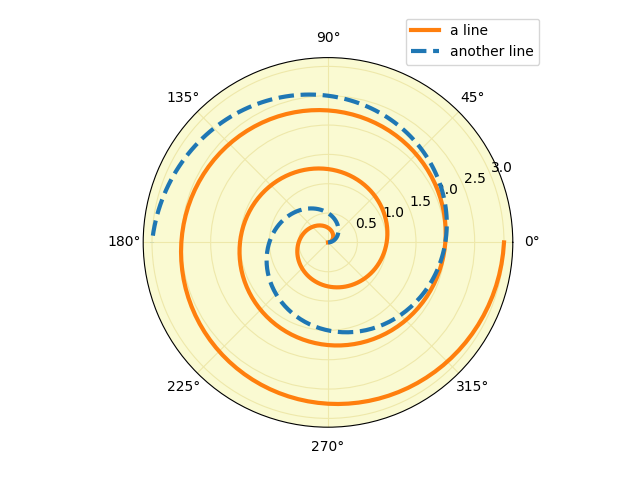# Polar Legend¶

Demo of a legend on a polar-axis plot.

import matplotlib.pyplot as plt
import numpy as np

fig = plt.figure()

r = np.linspace(0, 3, 301)
theta = 2 * np.pi * r
ax.plot(theta, r, color="tab:orange", lw=3, label="a line")
ax.plot(0.5 * theta, r, color="tab:blue", ls="--", lw=3, label="another line")
ax.tick_params(grid_color="palegoldenrod")
# For polar axes, it may be useful to move the legend slightly away from the
# axes center, to avoid overlap between the legend and the axes.  The following
# snippet places the legend's lower left corner just outside of the polar axes
# at an angle of 67.5 degrees in polar coordinates.